# Fermi Dirac Distribution Function

Distribution functions are nothing but the probability density functions used to describe the probability with which a particular particle can occupy a particular energy level. When we speak of Fermi-Dirac distribution function, we are particularly interested in knowing the chance by which we can find a fermion in a particular energy state of an atom (more information on this can be found in the article “Atomic Energy States”). Here, by fermions, we mean the electrons of an atom which are the particles with ½ spin, bound to Pauli exclusion principle.

## Necessity of Fermi Dirac Distribution Function

In fields like electronics, one particular factor which is of prime importance is the conductivity of materials. This characteristic of the material is brought about the number of electrons which are free within the material to conduct electricity.

As per energy band theory (refer to the article “Energy Bands in Crystals” for more information), these are the number of electrons which constitute the conduction band of the material considered. Thus inorder to have an idea over the conduction mechanism, it is necessary to know the concentration of the carriers in the conduction band.

## Fermi Dirac Distribution Expression

Mathematically the probability of finding an electron in the energy state E at the temperature T is expressed asWhere,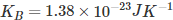is the Boltzmann constant
T is the absolute temperature
Ef is the Fermi level or the Fermi energy

Now, let us try to understand the meaning of Fermi level. In order to accomplish this, put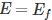in equation (1). By doing so, we get,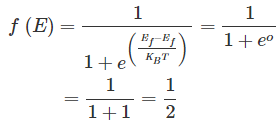This means the Fermi level is the level at which one can expect the electron to be present exactly 50% of the time.

### Fermi Level in Semiconductors

Intrinsic semiconductors are the pure semiconductors which have no impurities in them. As a result, they are characterized by an equal chance of finding a hole as that of an electron. This inturn implies that they have the Fermi-level exactly in between the conduction and the valence bands as shown by Figure 1a.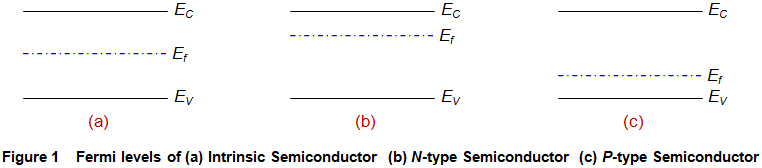Next, consider the case of an n-type semiconductor. Here, one can expect more number of electrons to be present in comparison to the holes. This means that there is a greater chance of finding an electron near to the conduction band than that of finding a hole in the valence band. Thus, these materials have their Fermi-level located nearer to conduction band as shown by Figure 1b.
Following on the same grounds, one can expect the Fermi-level in the case of p-type semiconductors to be present near the valence band (Figure 1c). This is because, these materials lack electrons i.e. they have more number of holes which makes the probability of finding a hole in the valence band more in comparison to that of finding an electron in the conduction band.

## Effect of temperature on Fermi-Dirac Distribution Function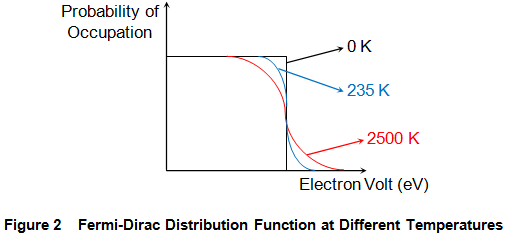At T = 0 K, the electrons will have low energy and thus occupy lower energy states. The highest energy state among these occupied states is referred to as Fermi-level. This inturn means that no energy states which lie above the Fermi-level are occupied by electrons. Thus we have a step function defining the Fermi-Dirac distribution function as shown by the black curve in Figure 2.
However as the temperature increases, the electrons gain more and more energy due to which they can even rise to the conduction band. Thus at higher temperatures, one cannot clearly distinguish between the occupied and the unoccupied states as indicated by the blue and the red curves shown in Figure 2.

Want To Learn Faster? 🎓
Get electrical articles delivered to your inbox every week.
No credit card required—it’s 100% free.